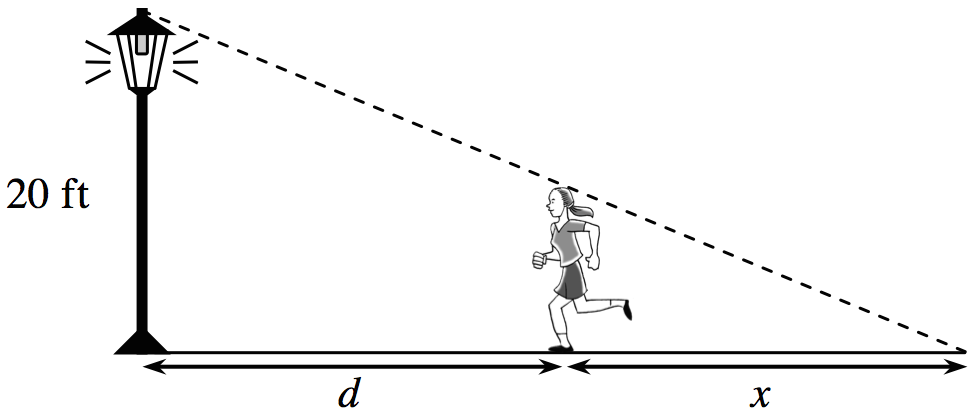Home > APCALC > Chapter 1 > Lesson 1.5.1 > Problem1-201

1-201.

Shehazana, who is $5.5$ feet tall, is walking toward a $20$-foot tall streetlight. Write an equation for the length of her shadow, $x$, in terms of the distance, $d$, she is from the pole. Visualize this situation using the .Do you see the similar right triangles in this diagram? Recall that similar triangles have proportional sides. Set up a proportion and solve for $x$.

$\frac{5.5}{20} = \frac{x}{d}$

Use the eTool below to visualize this situation.
Click the link at right for the full version of the eTool: Calc 1-201 HW eTool